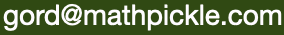Select Page

This is a single variable algebra puzzle in which students try to find squares of different sizes to tile a rectangle. I prefer the two variable algebra variant on the right.

Get students to create their own history puzzles.

#### Algebra on Rectangles

##### (R. L. Brooks, C. A. B. Smith, A. H. Stone and W. T. Tutte, 1930s)

Students use linear algebra to build rectangles out of squares. The results are aesthetically pleasing and difficult to reproduce by trial and error.

Lessons should start on graph paper - giving students the challenge of trying to "build a rectangle using squares of all different sizes." Students will find it extremely difficult. After several minutes hold a vote asking if it is an impossible problem.

PS. These puzzles are based on the work of Brooks, Smith, Stone and Tutte at Cambridge in the 1930s. Although this technique failed to produce a result, it can be retroactively used to create the best imaginable way to introduce algebra.

PPS. The power of introducing algebra by way of a problem so difficult that students cannot solve it through non-algebraic means is not to be underestimated.

#### Standards for Mathematical Practice

MathPickle puzzle and game designs engage a wide spectrum of student abilities while targeting the following Standards for Mathematical Practice:

##### MP1 Toughen up!

Students develop grit and resiliency in the face of nasty, thorny problems. It is the most sought after skill for our students.

##### MP2 Think abstractly!

Students take problems and reformat them mathematically. This is helpful because mathematics lets them use powerful operations like addition.

##### MP3 Work together!

Students discuss their strategies to collaboratively solve a problem and identify missteps in a failed solution. MathPickle recommends pairing up students for all its puzzles.

##### MP4 Model reality!

Students create a model that mimics the real world. Discoveries made by manipulating the model often hint at something in the real world.

##### MP5 Know the tools.

Students master the tools at their fingertips - whether it's a pencil or an online app.

##### MP6 Be precise!

Students learn to communicate using precise terminology. MathPickle encourages students not only to use the precise terms of others, but to invent and rigorously define their own terms.

##### MP7 Be observant!

Students learn to identify patterns. This is one of the things that the human brain does very well. We sometimes even identify patterns that don't really exist 😉

##### MP8 Be lazy!?!

Students learn to seek for shortcuts. Why would you want to add the numbers one through a hundred if you can find an easier way to do it?